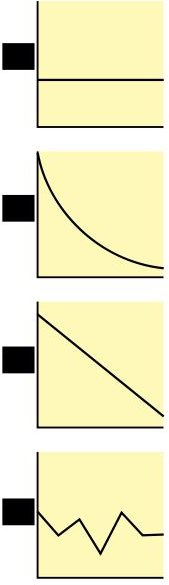# Example of Declining-Balance Depreciation Computation vs. Straight-Line Method

## What is the Declining Balance Depreciation?Declining balance depreciation is a method of allocating capitalized costs that uses the net book value (acquisition cost less accumulated depreciation) as the base amount for calculating the annual depreciation.

The depreciation cost is determined by using a percentage rate derived by dividing a year’s depreciation over the estimated useful life.

This may seem confusing to those who are not too familiar with the mechanics of depreciation; hence, we will explain the declining balance depreciation concept by way of explanation and examples.

## Example of Declining-Balance Depreciation Computation

Given: An office printer, purchased on January 1 at an acquisition cost of \$5,000 and an estimated useful life of 5 years — Assuming further that there is a scrap value of \$1,500 to be recognized, based on the company’s fixed assets capitalization policies

Using these givens, the depreciation under the declining balance depreciation method is computed as follows:

Calculate the Rate of Annual Depreciation:

The rate of annual depreciation is calculated by creating a fraction for 1 year’s depreciation over the 5 years of estimated useful life, or 1/5. This fraction will be converted into a decimal number by dividing 1 over 5, to get 0.20 and then multiplying it by 100% to arrive at its percentage equivalent. The formula for the rate of depreciation per year is as follows:

Rate of Annual Depreciation = 1/5 x 100% = 20%

Calculating the Annual Depreciation Costs:

In calculating the annual depreciation using this method, you have to refer to the running balance of the accumulated depreciation account in order to determine the base amount used for calculating the yearly depreciation costs.

• Depreciation for the 1st year =(\$5,000 – \$0.00) x 20% = \$1,000
• Depreciation for the 2nd year = (\$5,000 – \$1,000) x 20% = \$4,000 x 20% = \$800
• Depreciation for the 3rd year = (\$5,000 – \$1,800) = \$3,200 x 20% = \$640
• Depreciation for the 4th year = (\$5,000- \$2,440) = \$2,560 x 20% = \$512
• Depreciation for the 5th year = (\$5,000 – \$2,952) = \$2,048 x 20% = \$410
• Depreciation for the 6th year = (\$3,500 – \$3,362) = \$138

Note: The final year of depreciation was adjusted to arrive at the residual or scrap value of \$1,500.

Running Balance of Accumulated Depreciation Per Year:

• Accumulated Depreciation 1st year = \$0.00 + \$0.00 = \$0.00
• Accumulated Depreciation 2nd year = \$1,000 + \$800 = \$1,800
• Accumulated Depreciation 3rd year = \$1,800 + \$640 = \$2,440
• Accumulated Depreciation 4th year = \$2,440 + \$512 = \$2,952
• Accumulated Depreciation 5th year = \$2,952 + \$410 = \$3,362
• Accumulated Depreciation 6th year = \$3,362 + \$138 = \$3,500

## Explaining the Effects of the Declining-Balance Depreciation vs. Straight-Line Method

Take note that in the declining balance depreciation, the 1st year depreciation cost is \$1,000, which decreases as the years progress until the NBV reaches the scrap value amount.

If one is to use the straight-line method, the entire 5 years of estimated useful life will recognize a uniform depreciation cost of \$700. This is derived via the following computation:

Straight-line depreciation per year = (\$5,000 – \$1,500) / 5 years = \$700

Hence, the first two years of allocated cost using the declining balance method is \$1,800, which is higher than \$1,400 (\$700 x 2 years) if the straight-line method were used. The rationale for this computation is that the use of the asset is more extensive during the earlier years; hence, the allocated cost is higher.

As the fixed asset ages through the passing of its estimated useful life, the company incurs additional costs related to the use of said fixed asset, by way of repairs and maintenance costs. Thus, the burden of the allocated costs likewise decrease in later years because the fixed asset’s contribution is no longer as significant as it was in the earlier years of its estimated useful life.

However, based on the accounting study guide issued for US GAAP explanation, the modified 200% or 150% declining balance is more acceptable.

Readers can find the explanation and examples for the 200% Declining Balance Method in a separate article entitled “Double-Declining Depreciation: Explanation & Examples.”

## Reference Materials and Image Credit Section:

Reference :

• US GAAP Accounting Study Guide –https://accountinginfo.com/study/dep/depreciation-01.htm

Image Credit: